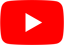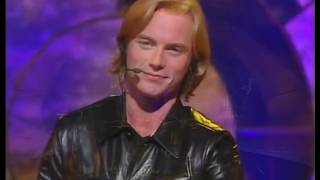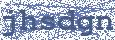Let The Message Run Free
Boyzone

## BOYZONE

Let The Message Run Free Lyrics

In this world of confusion
Under the same sun
Save us from complication
Let the message run
I was speaking so many tongues
In a voice loud and clear
We'll bridge communications
Let the message run
So do I have to talk to another?
Can we speak as one?
And if we talk to each other
We should all be
We should all be
We should all be one
One

Let us run free
Oh let us see (oh let us see)
and through the eyes of a child
Let the message run free (free)

Let us run free (let us run free)
Oh let us see (oh let us see)
Through the eyes of a child
Let the message run free
(let the message run, let the message run)

Through the wires like lightening
Over land and ocean, yeah
No more in isolation
We should all be
We should all be
We should all be one
One

Let us run free (let us run free)
Oh let us see (oh let us see)
Through the eyes of a child
Let the message run free
(let the message run, let the message run)

(Let's run free...)
Let us run free
(Oh, let us run free)
Oh let us see
(oh let us see)
Through the eyes of a child
Let the message run free
(let the message run, let the message run free)

Through the wires like lightening
Over land and ocean
No more in isolation
Let the message run free
Let the message run free

Let us run free
(let us run free)
Oh let us see
(oh let us see)
Through the eyes of a child
Let the message run freeHottest Lyrics with Videos
6778bf6c838a83f29878837413d26b9e